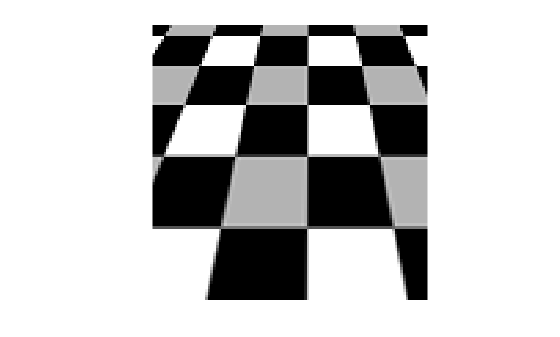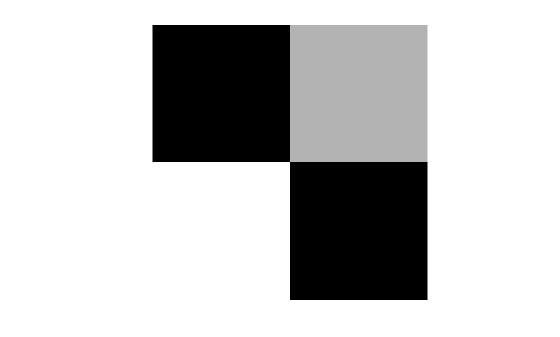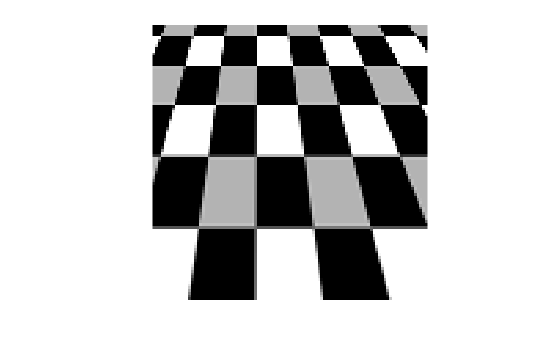# tformarray

Apply spatial transformation to N-D array

## Syntax

``B = tformarray(A,T,R,tdims_A,tdims_B,tsize_B,tmap_B,F)``

## Description

example

````B = tformarray(A,T,R,tdims_A,tdims_B,tsize_B,tmap_B,F)` applies a spatial transformation to array `A` to produce array `B`.```

## Examples

collapse all

Create a 2-by-2 square checkerboard image where each square is 20 pixels wide. Display the image.

```I = checkerboard(20,1,1); figure imshow(I)```Transform the checkerboard with a projective transformation. First create a spatial transformation structure.

```T = maketform('projective',[1 1; 41 1; 41 41; 1 41],... [5 5; 40 5; 35 30; -10 30]);```

Create a resampler. Use the pad method `'circular'` when creating the resampler, so that the output appears to be a perspective view of an infinite checkerboard.

`R = makeresampler('cubic','circular');`

Perform the transformation, specifying the transformation structure and the resampler. For this example, swap the output dimensions, and specify a 100-by-100 output image. Leave argument `tmap_B` empty since you specify argument `tsize_B`. Leave argument `F` empty since the fill value is not needed.

```J = tformarray(I,T,R,[1 2],[2 1],[100 100],[],[]); figure imshow(J)```Create a 2-by-2 square checkerboard image where each square is 20 pixels wide. Display the image.

```I = checkerboard(20,1,1); figure imshow(I)```Transform the checkerboard with a projective transformation. First create a spatial transformation structure.

```T = maketform('projective',[1 1; 41 1; 41 41; 1 41],... [5 5; 40 5; 35 30; -10 30]);```

Create a resampler. Use the pad method `'circular'` when creating the resampler, so that the output appears to be a perspective view of an infinite checkerboard.

`R = makeresampler('cubic','circular');`

Create arrays that specify the mapping of points from input space to output space. This example uses anisotropic sampling, where the distance between samples is larger in one direction than the other.

```samp_x = 1:1.5:150; samp_y = 1:100; [x,y] = meshgrid(samp_x,samp_y); tmap = cat(3,x,y); size(tmap)```
```ans = 1×3 100 100 2 ```

Note the size of `tmap`. The output image will have dimensions 100-by-100.

Perform the transformation, specifying the transformation structure and the resampler. Specify the output map as `tmap`. Leave argument `tsize_B` empty, since you specify argument `tmap_B`. The fill value does not matter since the resampler is circular.

```J = tformarray(I,T,R,[1 2],[1 2],[],tmap,[]); figure imshow(J)```The length of checkerboard squares is larger in the y-direction than in the x-direction, which agrees with the larger sampling distance between points in the vector `samp_x`. Compared to the result using isotopic point mapping (see example Transform Checkerboard Image), three additional columns of the checkerboard appear at the right of the transformed image, and no new rows are added to the transformed image.

## Input Arguments

collapse all

Input image, specified as a nonsparse numeric array. `A` can be real or complex.

Data Types: `single` | `double` | `int8` | `int16` | `int32` | `uint8` | `uint16` | `uint32` | `logical`
Complex Number Support: Yes

Spatial transformation, specified as a `TFORM` spatial transformation structure. You typically use the `maketform` function to create a `TFORM` structure.

`tformarray` uses `T` and the function `tforminv` to compute the corresponding location in the input transform subscript space for each location in the output transform subscript space. `tformarray` defines the input transform space by `tdims_B` and `tsize_B` and the output transform subscript space by `tdims_A` and `size(A)`.

If `T` is empty, then `tformarray` operates as a direct resampling function. Further, if `tmap_B` is:

• Not empty, then `tformarray` applies the resampler defined in `R` to compute values at each transform space location defined in `tmap_B`

• Empty, then `tformarray` applies the resampler at each location in the output transform subscript grid

Data Types: `struct`

Resampler, specified as a structure. A resampler structure defines how to interpolate values of the input array at specified locations. `R` is created with `makeresampler`, which allows fine control over how to interpolate along each dimension. `makeresampler` also controls what input array values to use when interpolating close to the edge of the array.

Data Types: `struct`

Input transform dimensions, specified as a row vector of finite, positive integers.

`tdims_A` and `tdims_B` indicate which dimensions of the input and output arrays are involved in the spatial transformation. Each element must be unique. The entries need not be listed in increasing order, but the order matters. The order specifies the precise correspondence between dimensions of arrays `A` and `B` and the input and output spaces of the transformation `T`.

`length(tdims_A)` must equal `T.ndims_in`, and `length(tdims_B)` must equal `T.ndims_out`.

For example, if `T` is a 2-D transformation, `tdims_A = [2 1]`, and ```tdims_B = [1 2]```, then the row and column dimensions of `A` correspond to the second and first transformation input-space dimensions, respectively. The row and column dimensions of `B` correspond to the first and second output-space dimensions, respectively.

Data Types: `double`

Output transform dimensions, specified as a row vector of finite, positive integers. For more information, see `tdims_A`.

Data Types: `double`

Size of the output array transform dimensions, specified as a row vector of finite, positive integers. The size of `B` along nontransform dimensions is taken directly from the size of `A` along those dimensions.

For example, if `T` is a 2-D transformation, `size(A) = [480 640 3 10]`, `tdims_B` is `[2 1]`, and `tsize_B` is `[300 200]`, then `size(B)` is `[200 300 3 10]`.

Data Types: `double`

Point locations in output space, specified as a nonsparse, finite real-valued array. `tmap_B` is an optional argument that provides an alternative way of specifying the correspondence between the position of elements of `B` and the location in output transform space. `tmap_B` can be used, for example, to compute the result of an image warp at a set of arbitrary locations in output space.

If `tmap_B` is not empty, then the size of `tmap_B` is

` [D1 D2 D3 ... DN L]`
where `N` equals `length(tdims_B)`. `tsize_B` should be `[]`.

The value of `L` depends on whether `T` is empty. If `T` is:

• Not empty, then `L` is `T.ndims_out`, and each L-dimension point in `tmap_B` is transformed to an input-space location using `T`

• Empty, then `L` is `length(tdims_A)`, and each `L`-dimensional point in `tmap_B` is used directly as a location in input space.

Data Types: `double`

Fill values, specified as a numeric array or scalar. The fill values in `F` can be used in three situations:

• When a separable resampler is created with `makeresampler` and its `padmethod` is set to either `'fill'` or `'bound'`.

• When a custom resampler is used that supports the `'fill'` or `'bound'` pad methods (with behavior that is specific to the customization).

• When the map from the transform dimensions of `B` to the transform dimensions of `A` is deliberately undefined for some points. Such points are encoded in the input transform space by `NaN`s in either `tmap_B` or in the output of `tforminv`.

In the first two cases, fill values are used to compute values for output locations that map outside or near the edges of the input array. Fill values are copied into `B` when output locations map well outside the input array. See `makeresampler` for more information about `'fill'` and `'bound'`.

When `F` is:

• A scalar (including `NaN`), its value is replicated across all the nontransform dimensions.

• Nonscalar, its size depends on `size(A)` in the nontransform dimensions. Specifically, if `K` is the `J`th nontransform dimension of `A`, then `size(F,J)` must be either `size(A,K)` or `1`. As a convenience, `tformarray` replicates `F` across any dimensions with unit size such that after the replication `size(F,J)` equals `size(A,K)`.

For example, suppose `A` represents 10 RGB images and has size 200-by-200-by-3-by-10, `T` is a 2-D transformation, and `tdims_A` and `tdims_B` are both [1 2]. In other words, `tformarray` applies the same 2-D transform to each color plane of each of the 10 RGB images. In this situation you have several options for `F`:

• `F` can be a scalar, in which case the same fill value is used for each color plane of all 10 images.

• `F` can be a 3-by-1 vector, ```[R G B]'```. `tformarray` uses the RGB value as the fill value for the corresponding color planes of each of the 10 images.

• `F` can be a 1-by-10 vector. `tformarray` uses a different fill value for each of 10 images, with that fill value being used for all three color planes.

• `F` can be a 3-by-10 matrix. `tformarray` uses a different RGB fill color for each of the 10 images.

Data Types: `double`

## Output Arguments

collapse all

Transformed image, returned as a numeric array.